Bagging, Random Forest, and Boosting
library(ISLR)
library(tidyverse)
library(randomForest)
library(gbm)
library(MASS)
library(rpart)

## Bagging

### Motivation:

Decision trees discussed previously have high variance. Imagine we split the data into two parts at random and fit a decision tree to both halves. The results could be extremely different. What we want to do is find a procedure with low variance that will give similar results when applied over and over again.

data = read.csv("50_Startups.csv")
head(data)
set.seed(1)
split = sample (1:nrow(Boston), nrow(Boston)/2)
regressor2 = rpart(formula = Profit ~ R.D.Spend, data= data, subset = split, control = rpart.control(minsplit = 2))
xgrid = seq(min(data$R.D.Spend),max(data$R.D.Spend),1)
ggplot() +
geom_point(aes(x = data$R.D.Spend, y = data$Profit), color = 'red')+
geom_line(aes(x = xgrid, y = predict(regressor2, newdata = data.frame(R.D.Spend = xgrid))), color = 'blue')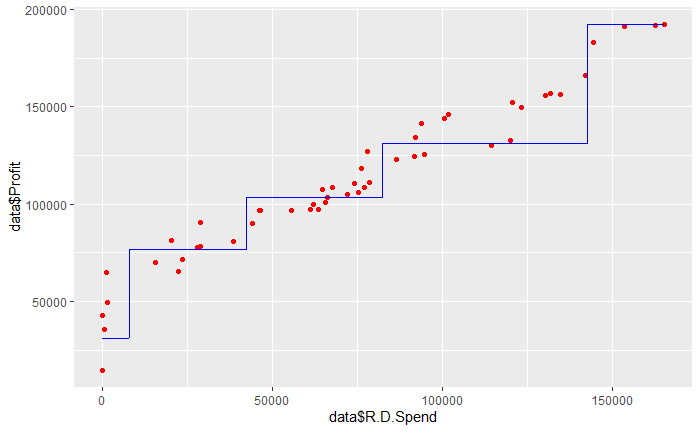set.seed(1)
split = sample (1:nrow(Boston), nrow(Boston)/2)
regressor2 = rpart(formula = Profit ~ R.D.Spend, data= data, subset = -split, control = rpart.control(minsplit = 2))
xgrid = seq(min(data$R.D.Spend),max(data$R.D.Spend),1)
ggplot() +
geom_point(aes(x = data$R.D.Spend, y = data$Profit), color = 'red')+
geom_line(aes(x = xgrid, y = predict(regressor2, newdata = data.frame(R.D.Spend = xgrid))), color = 'blue')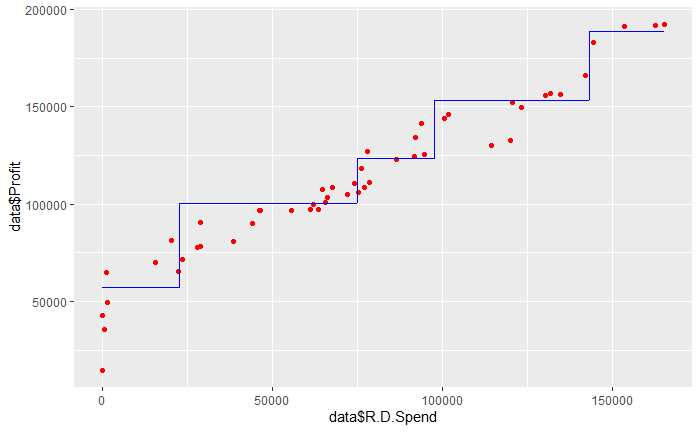Bagging also known as bootstrap aggregation will reduce the variance of a statistical learning method.

Ignoring the math for a moment, consider the the following intuition; Averaging a set of observations reduces the variance, so we could reduce the variance and increase the accuracy of our decision tree by taking many training sets from the population, building distinct trees, and averaging the results to obtain a single low variance model.

Unfortunately, we don’t expect to have access to multiple training sets. We can, however, bootstrap and take repeated samples from the single training set. This is called Bagging and works for lots of different statistical learning methods, but is especially useful for decision trees. Our procedure to implement this method begins by constructing B regression trees using B bootstrapped training sets and then averaging the results.

This gives us the following equation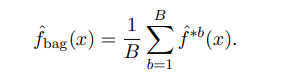Note These trees should be grown deep and not be pruned, so an individual tree should have high variance and low bias.

### Out of Bag Error Estimation (OOB)

We don’t need to cross validate OR use the validation set approach! Instead, we can use the Out of Bag Error Estimate

Recall that the key to bagging is that trees are repeatedly fit to bootstrapped subsets of the observations and that, as Dr. White mentioned in his bootstrap lecture, each bagged tree will only use about 2/3rds of the observations. The remaining one third of the observations for a given bagged tree are referred to as the out of bag observations.

We can predict the response for the ith observation using each of the trees in which that observation was OOB. This will yield around B/3 predictions for the ith observation. Then, we can find a single prediction by averaging these predictions or take a majority vote. This ultimately leads to an overall OOB MSE which is a valid estimate of the test error for the bagged model.

### Application of Bagging

Now to a good example on the Boston dataset

Training our Model

set.seed(12)
train = sample (1:nrow(Boston), nrow(Boston)/2)
bag.boston = randomForest(medv~., data = Boston, subset = train, mtry = 13, importance = TRUE)
# mtry -    Number of variables randomly sampled as candidates at each split. Note that the default values are different for classification (sqrt(p) where p is number of variables in x) and regression (p/3)
bag.boston

Call:
randomForest(formula = medv ~ ., data = Boston, mtry = 13, importance = TRUE,      subset = train)
Type of random forest: regression
Number of trees: 500
No. of variables tried at each split: 13

Mean of squared residuals: 14.09328
% Var explained: 84.14

Testing our Model

The OOB estimate for MSE is 14.09

yhat.bag = predict (bag.boston , newdata=Boston[-train ,])
ggplot()+
geom_point(aes(x = yhat.bag, y = Boston[-train, "medv"]))+
geom_abline(color = "red")+
xlab("Predicted Values")+
ylab("True Values")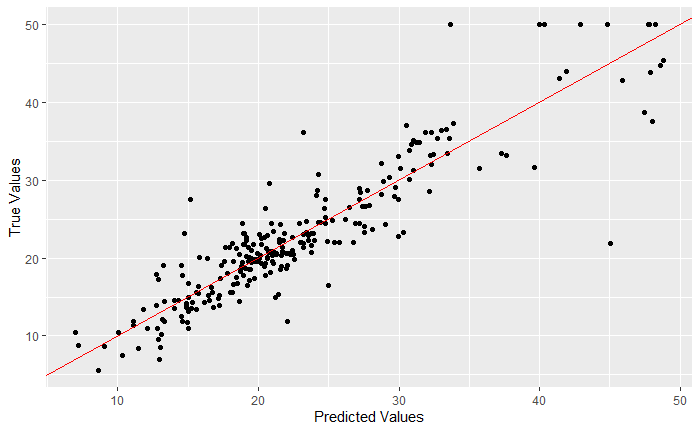mean((yhat.bag -Boston[-train, "medv"])^2)
 14.08248

The test set MSE associated with the bagged regression tree is 14.08, this is an improvement over the textbook example of a single optimally pruned tree.

set.seed(1)
bag.boston2 = randomForest(medv~., data = Boston, subset = train, mtry = 13, importance = TRUE, ntree = 1000)
yhat.bag2 = predict (bag.boston2 , newdata=Boston[-train ,])
mean((yhat.bag2 -Boston[-train, "medv"])^2)
 14.06875

### Interpretation

It’s not great. When we bag a large number of trees, it is no longer possible to represent the model with a single tree and it is no longer clear which variables are most important to the precedure.

Bagging improves the accuracy at the expense of interpretability

We do have some tools to identify importance though, we can use the RSS for regression trees or Gini index (Probability that a feature is classified incorrectly when selected randomly) for classification.

For regression - We can look at the total amount the RSS (Sum of Squared Residuals if you, like me, forgot) has decreased due to splits over a given predictor.

For classification - We can do the same with the Gini index measure.

## Random Forest

This is an improvement over bagged trees by decorrelating the trees. We still build multiple decision trees on bootstrapped training samples, but instead of using all predictors, we use only a random sample from the full set of predictors. Typically we choose the number of predictors to be approximately equal to the square root of the total number of predictors.

Weird but works! Why wouldn’t we want the trees to be built on all available data? How does this decorrelate the trees?

From the textbook “Suppose that there is one very strong predictor in the data set, along with a number of other moderately strong predictors. Then in the collection of bagged trees, most or all of the trees will use this strong predictor in the top split. Consequently, all of the bagged trees will look quite similar to each other. Hence the predictions from the bagged trees will be highly correlated.”

We know that averaging over highly correlated values doesn’t reduce the variance as much as doing so with uncorrelated values. This means that bagging doesn’t reduce the variance as much as we would like.

Random forest deals with this by forcing each tree to only consider a subset of the predictors. In the textbook example, this leads to some trees not even considering the strong predictor.

Main difference between random forest and bagging is the choise of predictor subset size

This leads to a reduction in both test error and OOB error over bagging. Basically use randomforest, not bagging.

Note Sometimes we may want to use less than the suggested number of predictors when variables are highly correlated. The randomForest library in R defaults to p/3 for regression trees.

### Application of Randomforest

set.seed(1)
rf.boston= randomForest(medv ~ .,data=Boston, subset=train, mtry=6, importance =TRUE)
yhat.rf = predict(rf.boston ,newdata=Boston[- train ,])
mean((yhat.rf-Boston[-train, "medv"])^2)
 12.94768

Wow! Look at how much better that is. Let’s see if we can understand what predictors are important.

importance(rf.boston)
          %IncMSE IncNodePurity
crim    14.162597    1495.43316
zn       2.354257      57.80273
indus    7.026734     721.89977
chas     4.271671      84.36145
nox     13.310095     907.93589
rm      34.210395    7223.81670
age      7.855808     317.85702
dis     13.120051    1058.37980
tax      8.696159     641.37864
ptratio 14.993781    1405.86289
black    5.980337     264.13753
lstat   31.040048    7704.31207

We see the %increase in MSE which is a measure of the decrease in accuracy in prediction on the OOB samples when a given variable is excluded.

We also see the total decrease in node impurity that results from splits over that variable (averaged over all trees.) For regression that is measured over RSS and for classification by deviance.

We can plot this!

varImpPlot(rf.boston)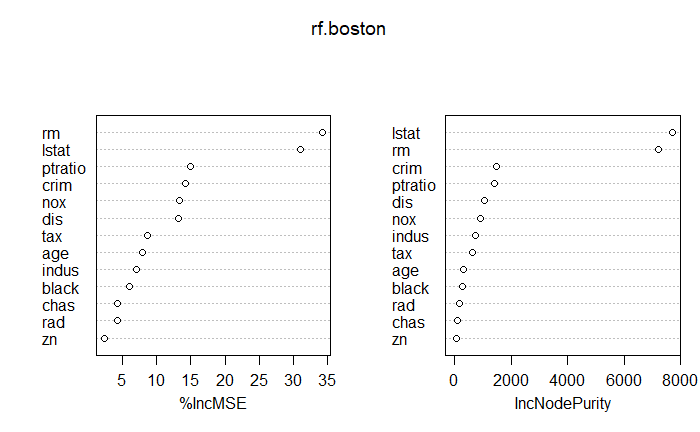## Boosting

Boosting is another general approach we can use to improve statistical learning methods. Boosting works like bagging does with multiple trees, but doesn’t use boot strapping and instead each tree is fit on a modified version of the original data. The main difference is that in boosting trees are grown sequentially based on information learned in previous trees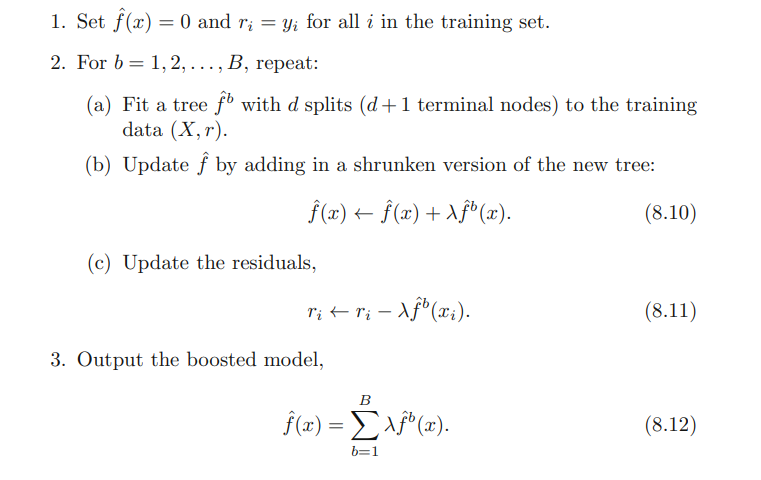• Unlike fitting a single large decision tree to the data, which amounts to fitting the data hard and potentially overfitting, the boosting approach instead learns slowly.

• Given the current model, we fit a decision tree to the residuals from the model. We then add this new decision tree into the fitted function in order to update the residuals.

• Each of these trees can be rather small, with just a few terminal nodes, determined by the parameter d in the algorithm.

• By fitting small trees to the residuals, we slowly improve f in areas where it does not perform well. The shrinkage parameter λ slows the process down even further, allowing more and different shaped trees to attack the residuals.

### Tuning Parameters for Boosting

1. The number of trees B. Unlike bagging and random forests, boosting can overfit if B is too large, although this overfitting tends to occur slowly if at all. We use cross-validation to select B.

2. The shrinkage parameter λ, a small positive number. This controls the rate at which boosting learns. Typical values are 0.01 or 0.001, and the right choice can depend on the problem. Very small λ can require using a very large value of B in order to achieve good performance.

3. The number of splits d in each tree, which controls the complexity of the boosted ensemble. Often d = 1 works well, in which case each tree is a stump, consisting of a single split and resulting in an additive model. More generally d is the interaction depth, and controls the interaction order of the boosted model, since d splits can involve at most d variables.

### Application

set.seed(12)
boost.boston=gbm(medv~.,data=Boston[train ,], distribution="gaussian",n.trees=5000, interaction.depth=4)
summary(boost.boston)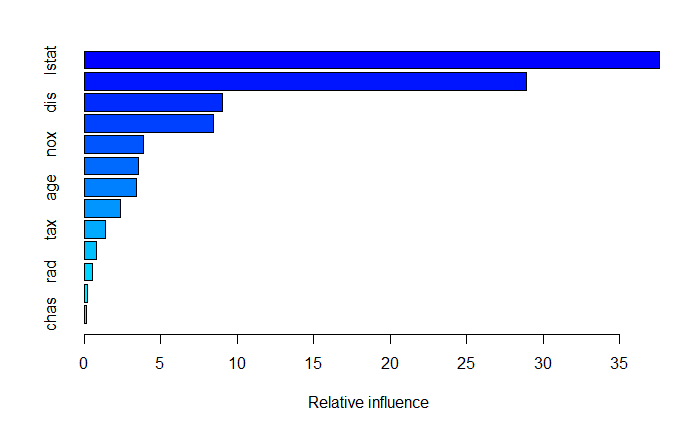Clearly rm and lstat are the most influential, lets take a look at them individually

plot(boost.boston ,i="rm")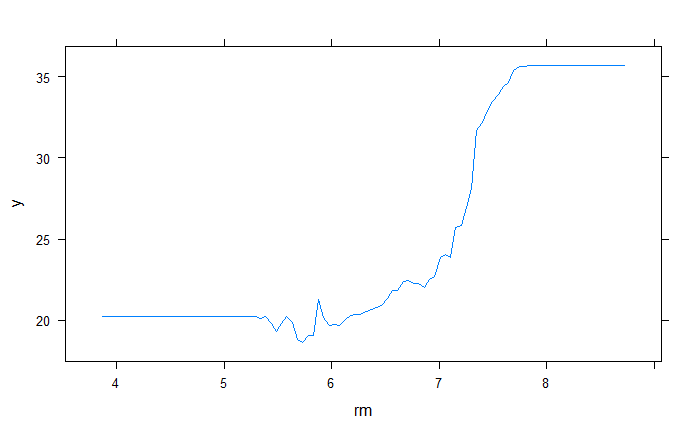plot(boost.boston ,i="lstat")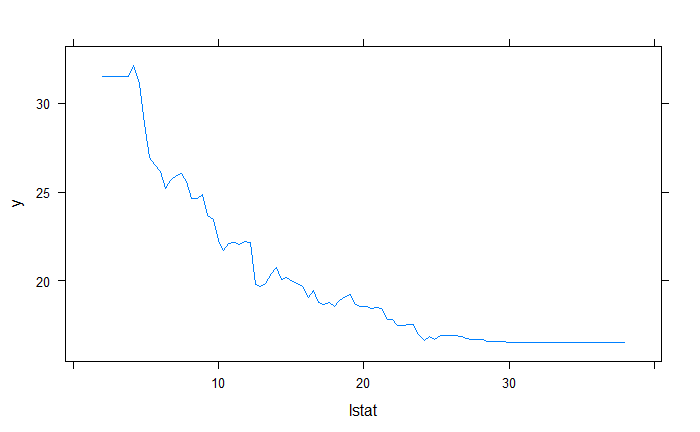yhat.boost=predict (boost.boston ,newdata =Boston[-train ,])#, n.trees=??)
Using 5000 trees...
best.iter <- gbm.perf(boost.boston, method = "OOB", plot = FALSE)
OOB generally underestimates the optimal number of iterations although predictive performance is reasonably competitive. Using cv_folds>1 when calling gbm usually results in improved predictive performance.
print(best.iter)
 102
attr(,"smoother")
Call:
loess(formula = object$oobag.improve ~ x, enp.target = min(max(4, length(x)/10), 50)) Number of Observations: 5000 Equivalent Number of Parameters: 39.99 Residual Standard Error: 0.2303  set.seed(1) yhat.boost=predict (boost.boston ,newdata =Boston[-train ,], n.trees=103) mean((yhat.boost-Boston[-train, "medv"])^2)  15.88817 Not a great MSE, but still better than bagging. Let’s try to tune it with lambda set.seed(1) boost.boston2=gbm(medv~.,data=Boston[train ,], distribution="gaussian",n.trees=5000, interaction.depth=5, shrinkage =.1) best.iter <- gbm.perf(boost.boston2, method = "OOB",plot = FALSE) OOB generally underestimates the optimal number of iterations although predictive performance is reasonably competitive. Using cv_folds>1 when calling gbm usually results in improved predictive performance. yhat.boost2=predict (boost.boston2 ,newdata =Boston[-train ,], n.trees=best.iter) mean((yhat.boost2-Boston[-train, "medv"])^2)  12.95806 An improvement! ##Classification data = read.csv("Social_Network_Ads.csv") head(data) set.seed(1234) data$Age=scale(data$Age) data$EstimatedSalary=scale(data$EstimatedSalary) train = sample (1:nrow(data), nrow(data)/2) classifier = randomForest(factor(Purchased)~EstimatedSalary + Age, data = data, subset= train, ntree = 10) yhat = predict(classifier, newdata=data[-train ,]) confusion_matrix = table(data[-train, 5],yhat) confusion_matrix  yhat 0 1 0 109 14 1 11 66 test = data[-train, ] X1 = seq(min(test$EstimatedSalary) - 1, max(test$EstimatedSalary) + 1, by = 0.1) X2 = seq(min(test$Age) - 1, max(test$Age) + 1, by = 0.1) grid = expand.grid(X1,X2) colnames(grid) = c("EstimatedSalary","Age") prob = predict(classifier, type = "response", newdata = grid) ggplot()+ geom_point(data = grid, aes(x=EstimatedSalary,y=Age, color = prob), size = .1)+ geom_point(aes(x=EstimatedSalary, y = Age, color= factor(Purchased)), data = test)+ geom_contour(aes(x=grid$EstimatedSalary, y = grid\$Age, z=as.numeric(prob)))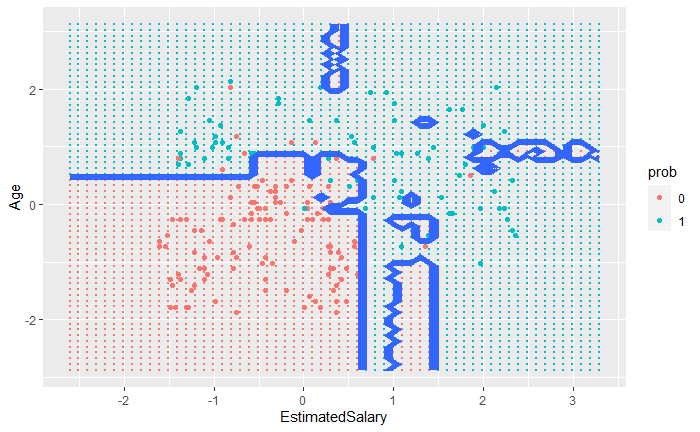##HW

1. Repeat the previous classification example using bagging and boosting methods and comment on any differences you observe.